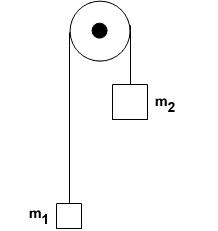# How to define kinetic energy of a compound system

A system is defined as two connected masses in an atwood machine and the earth. How do I find total kinetic energy in a system where more than one object is moving (or is only one object actually moving since the two masses are connected with a string? idk)This is an isolated system so... ΔK + ΔU = 0

How do I define energies of a system if there are multiple moving parts, each with its own energy? This is at the core of my confusion. I know that initially, there are no particles in this system with velocity, so Ki must be 0.
These are my questions

1.) How would you define the final kinetic energy? Do you treat the two moving blocks as a singly block instead of 2 separate blocks?

2.) What if a system consisted of 2 non connected blocks. How would you define final kinetic energy then?